# RD Sharma Solutions For Class 12 Maths Exercise 10.1 Chapter 10 Differentiability

RD Sharma Solutions for Class 12 Maths Exercise 10.1 Chapter 10 Differentiability are available at BYJU’S website in PDF format with solutions prepared by experienced faculty in a precise manner. It can mainly be used by the students as a study material to clear the Class 12 exams with a good score. Exercise 10.1 of the tenth chapter contains problems, which are solved based on the differentiability of a given function.

The RD Sharma Solutions are provided in an explanatory manner prepared by BYJU’S experts in Maths and are in accordance with the CBSE syllabus. The PDF of RD Sharma Solutions for Class 12 Maths Exercise 10.1 of Chapter 10 are provided here.

## RD Sharma Solutions For Class 12 Chapter 10 Differentiability Exercise 10.1:-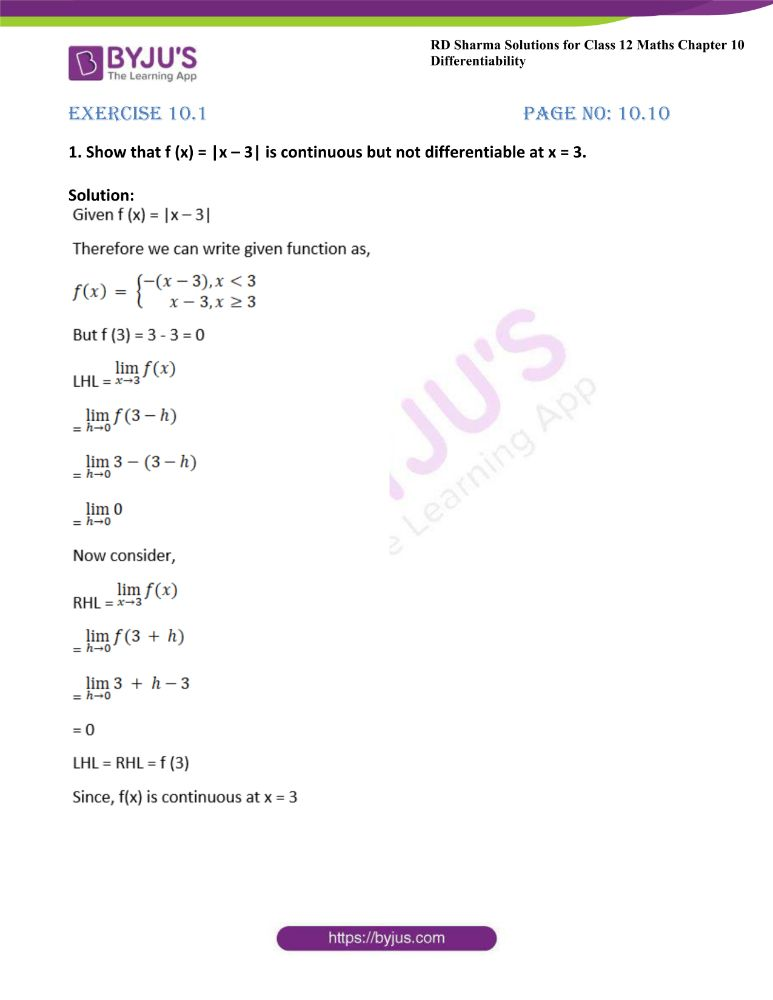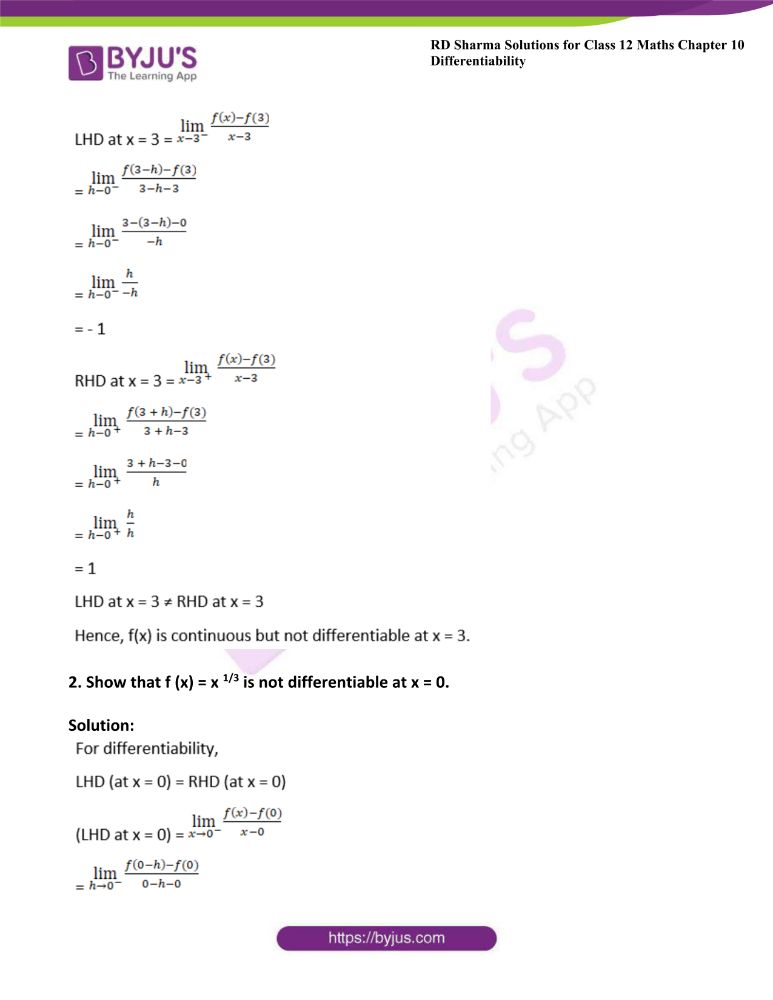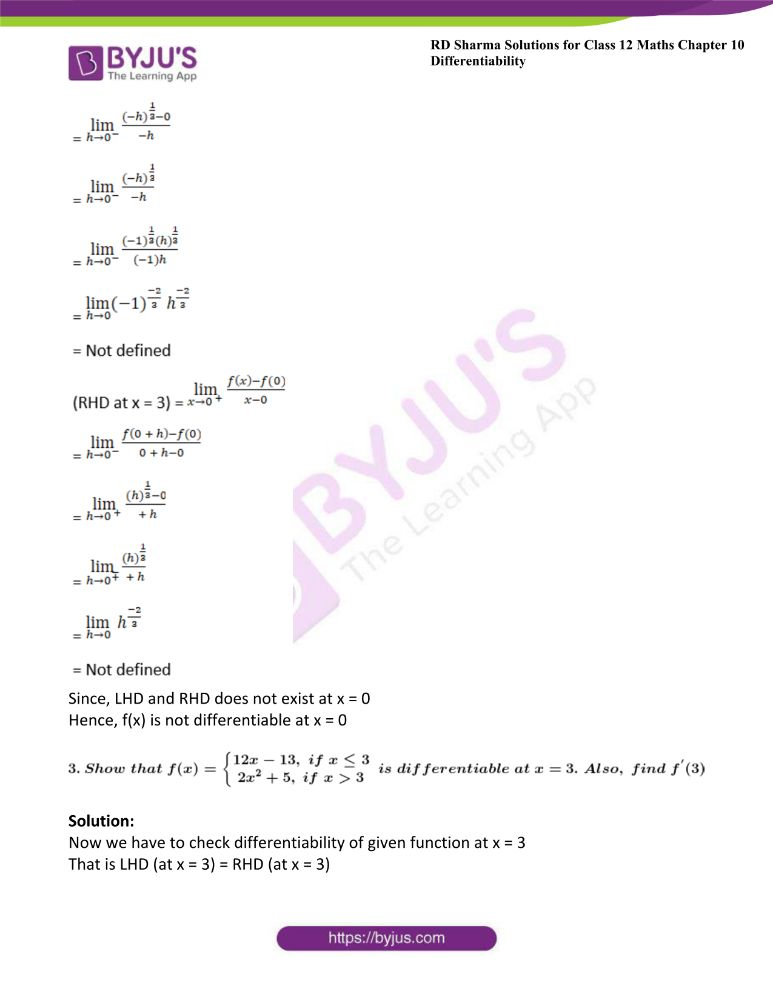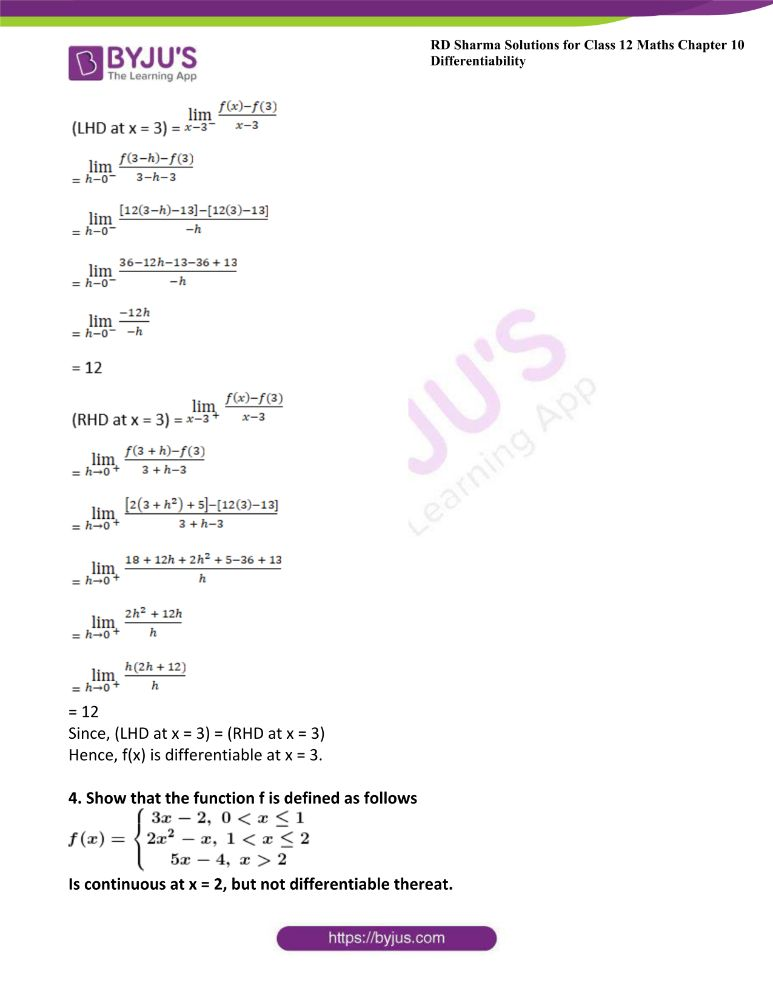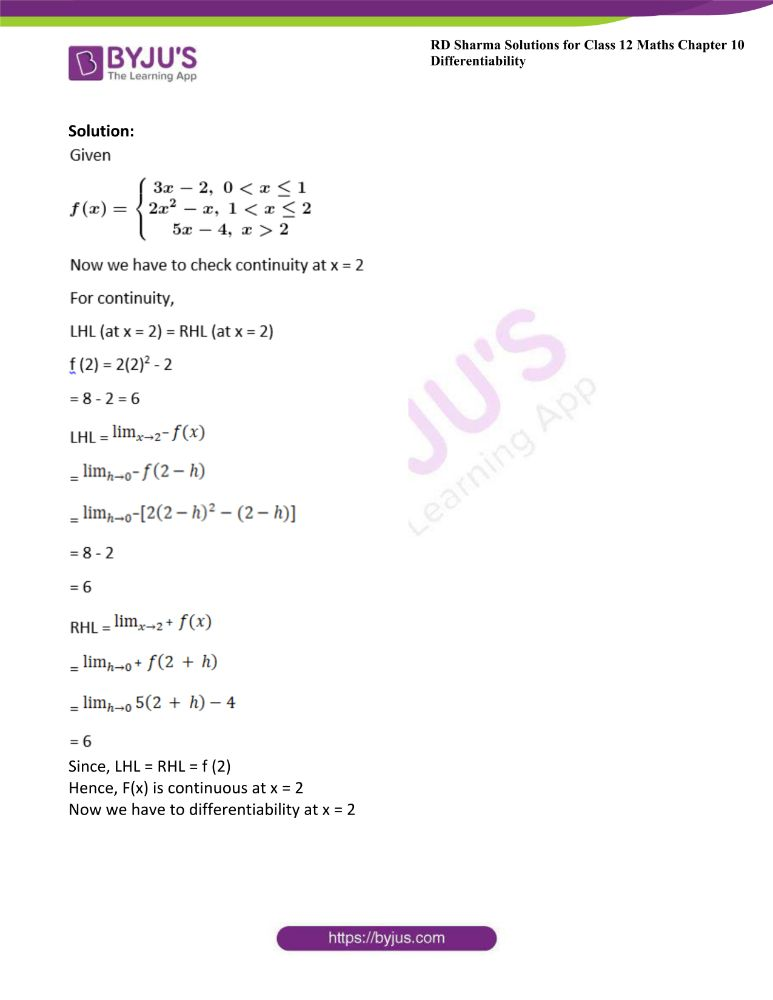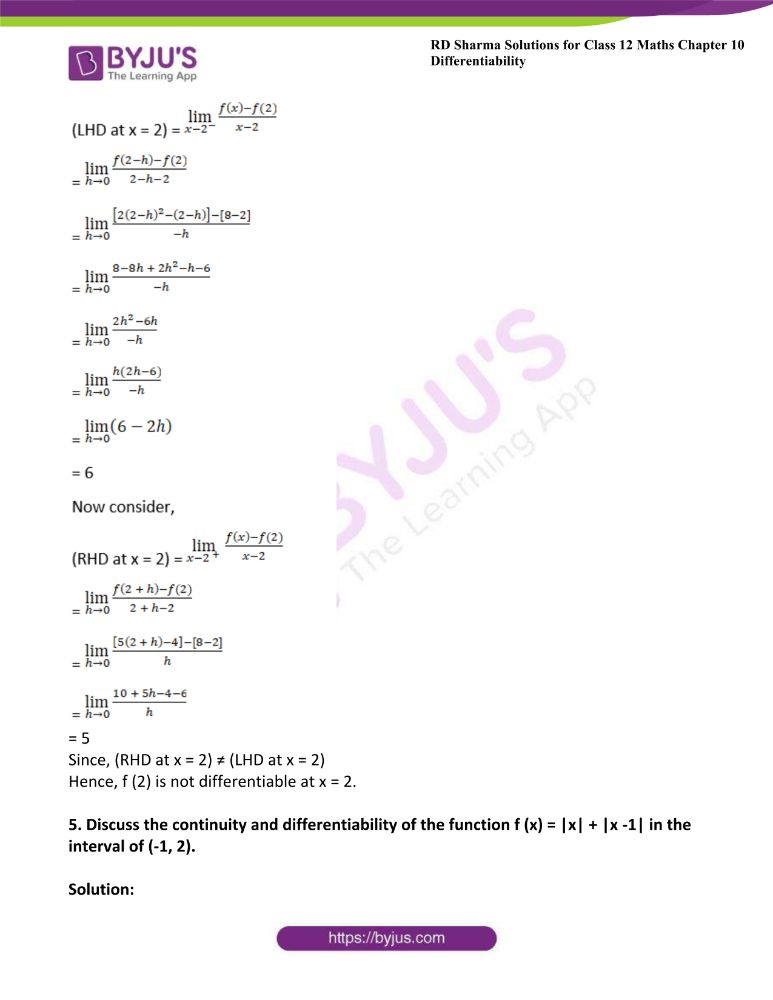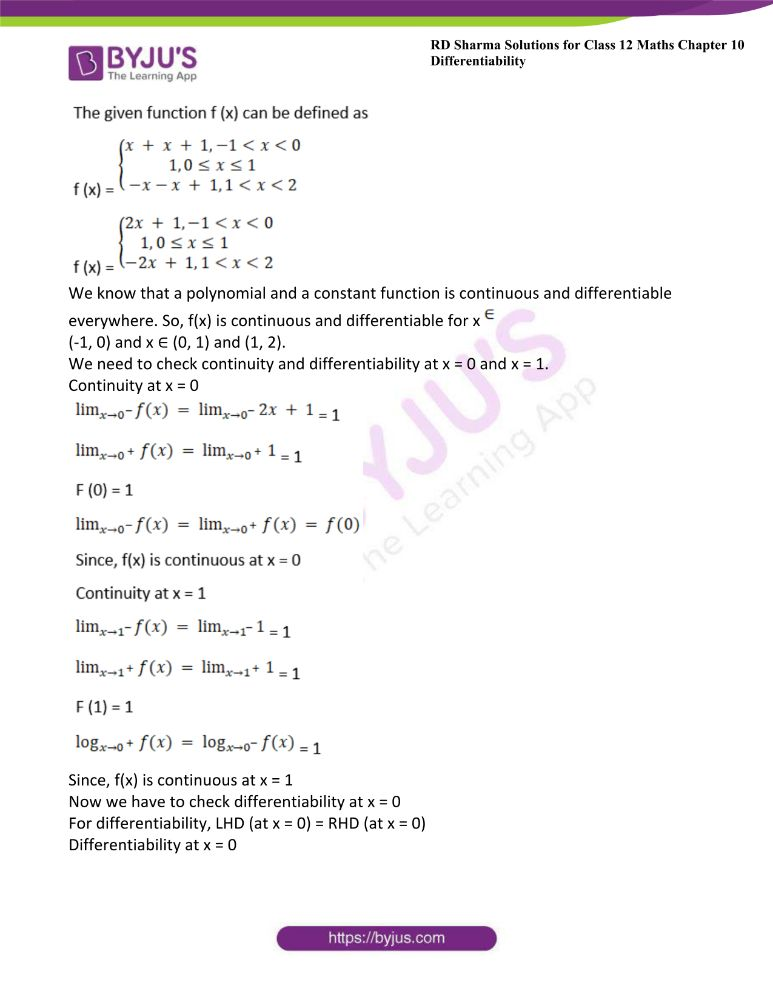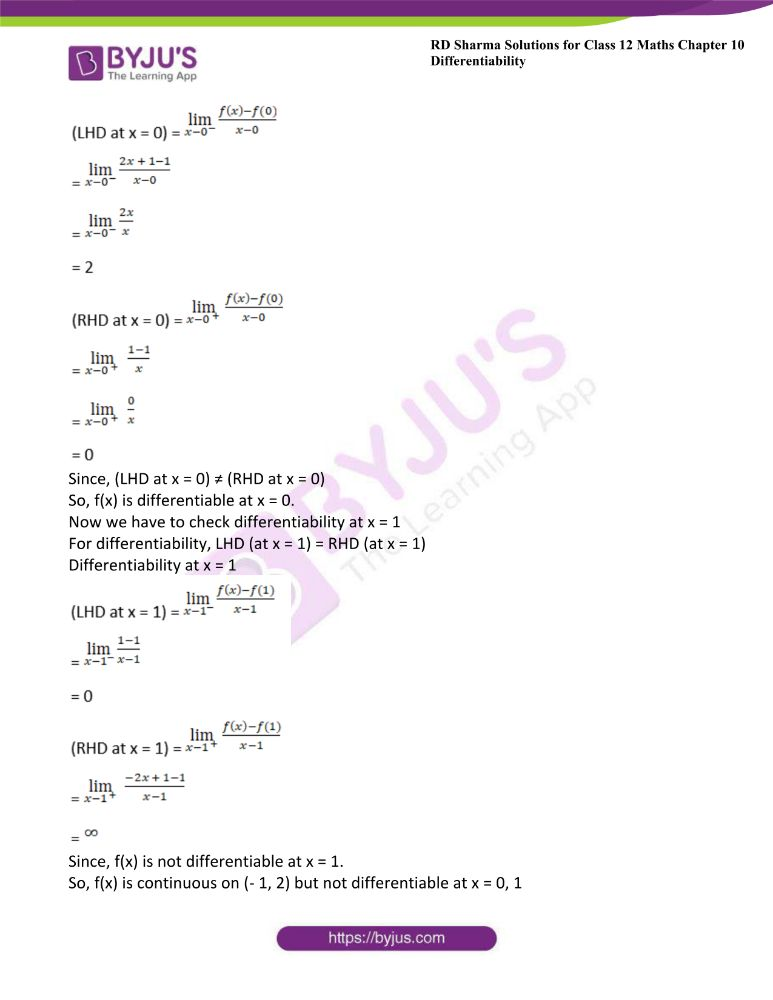### Access another exercise of RD Sharma Solutions For Class 12 Chapter 10 – Differentiability

Exercise 10.2 Solutions

### Access answers to Maths RD Sharma Solutions For Class 12 Chapter 10 – Differentiability Exercise 10.1

Exercise 10.1 Page No: 10.10

1. Show that f (x) = |x – 3| is continuous but not differentiable at x = 3.

Solution: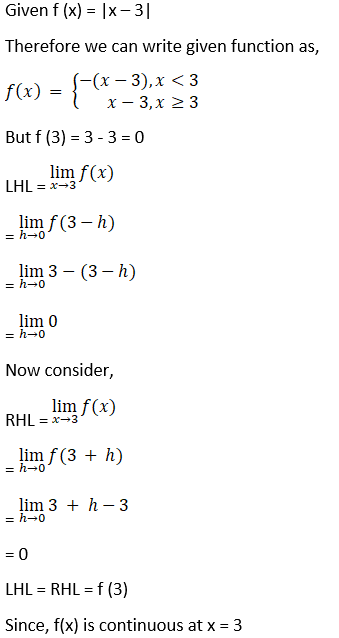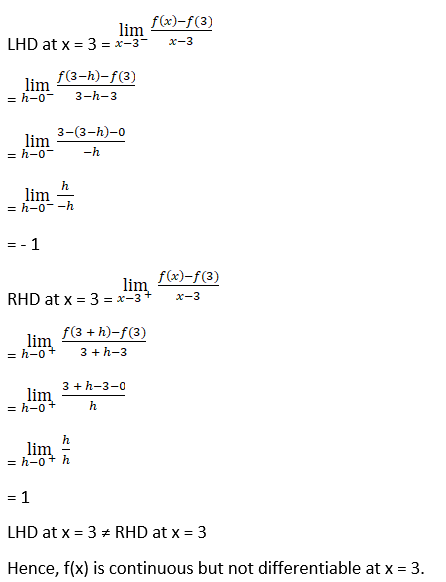2. Show that f (x) = x 1/3 is not differentiable at x = 0.

Solution: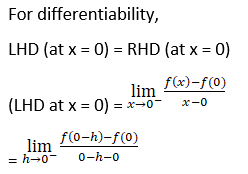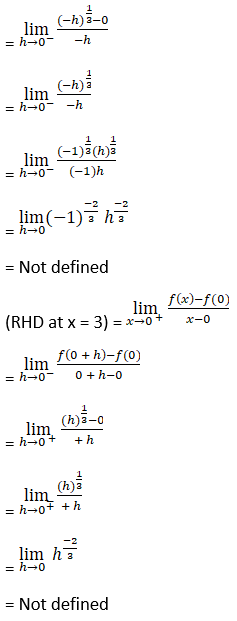Since, LHD and RHD does not exist at x = 0

Hence, f(x) is not differentiable at x = 0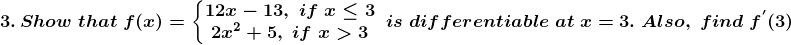Solution:

Now we have to check differentiability of given function at x = 3

That is LHD (at x = 3) = RHD (at x = 3)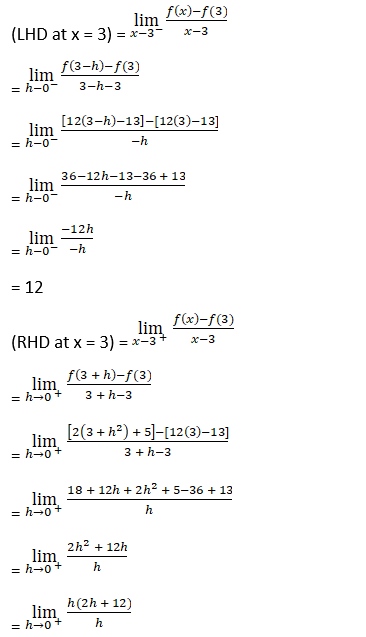= 12

Since, (LHD at x = 3) = (RHD at x = 3)

Hence, f(x) is differentiable at x = 3.

4. Show that the function f is defined as follows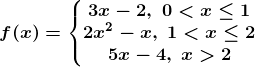Is continuous at x = 2, but not differentiable thereat.

Solution: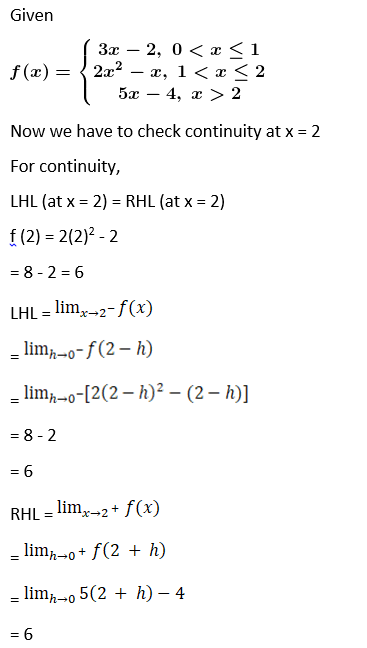Since, LHL = RHL = f (2)

Hence, F(x) is continuous at x = 2

Now we have to differentiability at x = 2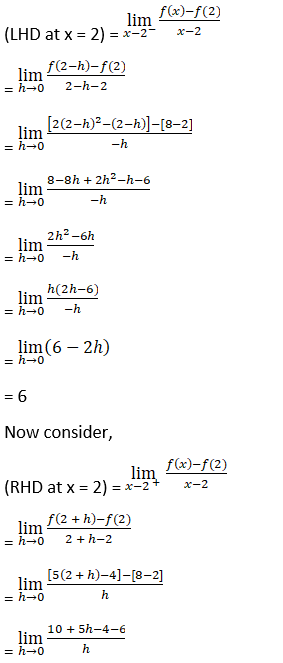= 5

Since, (RHD at x = 2) ≠ (LHD at x = 2)

Hence, f (2) is not differentiable at x = 2.

5. Discuss the continuity and differentiability of the function f (x) = |x| + |x -1| in the interval of (-1, 2).

Solution: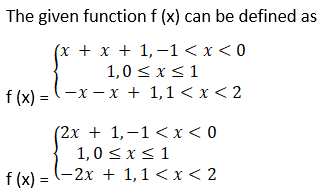We know that a polynomial and a constant function is continuous and differentiable everywhere. So, f(x) is continuous and differentiable for x ∈

(-1, 0) and x ∈ (0, 1) and (1, 2).

We need to check continuity and differentiability at x = 0 and x = 1.

Continuity at x = 0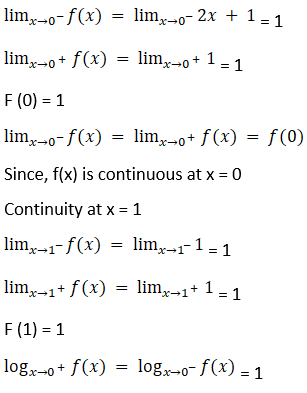Since, f(x) is continuous at x = 1

Now we have to check differentiability at x = 0

For differentiability, LHD (at x = 0) = RHD (at x = 0)

Differentiability at x = 0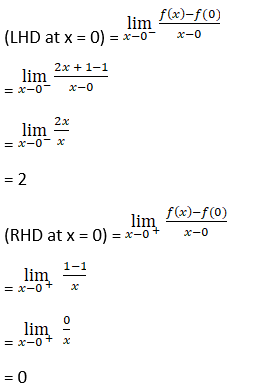Since, (LHD at x = 0) ≠ (RHD at x = 0)

So, f(x) is differentiable at x = 0.

Now we have to check differentiability at x = 1

For differentiability, LHD (at x = 1) = RHD (at x = 1)

Differentiability at x = 1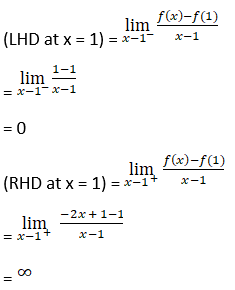Since, f(x) is not differentiable at x = 1.

So, f(x) is continuous on (- 1, 2) but not differentiable at x = 0, 1